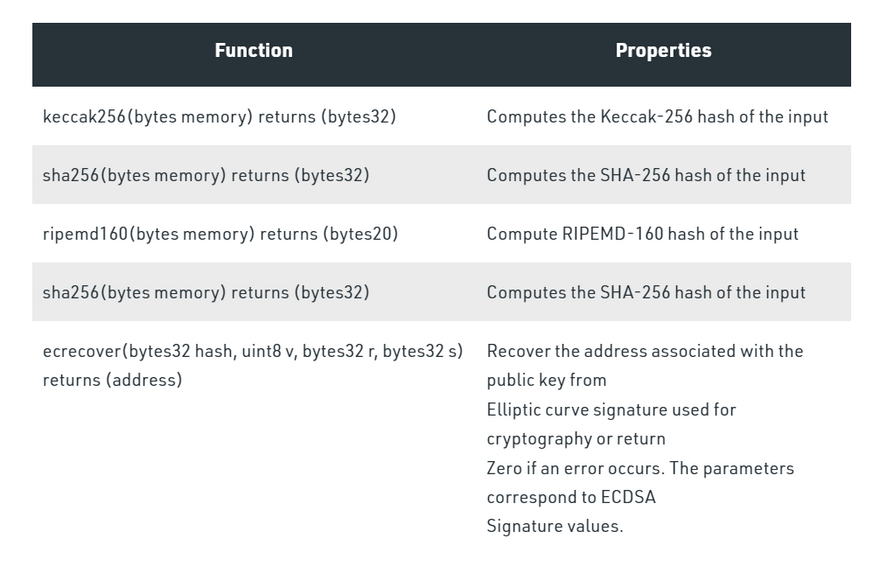## Developers Forum for XinFin XDC Network is a community of 2,074 amazing users

Developers Forum for XinFin XDC Network# What is Hashing in Solidity?

A cryptographic hash function is an algorithm that takes an arbitrary amount of data as input and produces the enciphered text of fixed size. Even a slight change in the input gives a completely different output.

Solidity provides the following cryptographic functions:Ethereum uses Keccak for hashing which is similar but not the same as SHA_256. For proof of work, it uses a custom scheme called ethash which is designed to be ASIC-resistant.

Example: In the below example, a smart contract is created to take a string as input and give an 8 digit hash as an output.

``````// pragma version
pragma solidity ^0.6.6;

// Creating a contract
contract helloGeeks
{
// We want hash to be of 8 digits
// hence we store 10^8 which is
// used to extract first 8 digits
// later by Modulus
uint hashDigits = 8;

// Equivalent to 10^8 = 8
uint hashModulus = 10 ** hashDigits;

// Function to generate the hash value
function _generateRandom(string memory _str)
public view returns (uint)
{
// "packing" the string into bytes and
// then applying the hash function.
// This is then typecasted into uint.
uint random =
uint(keccak256(abi.encodePacked(_str)));

// Returning the generated hash value
return random % hashModulus;
}

}
``````

Input:
`GeeksForGeeks`